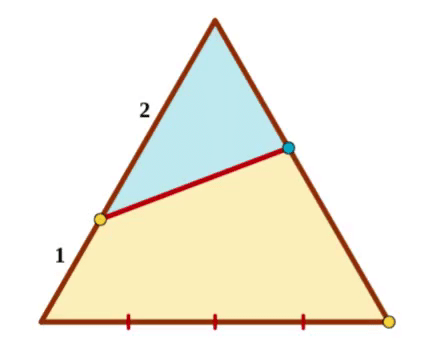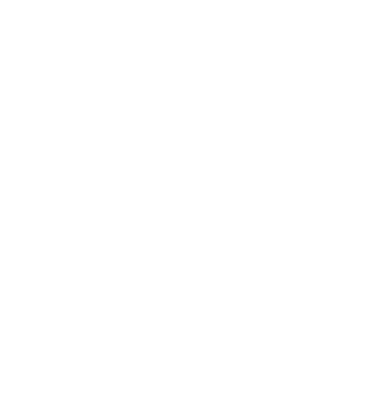Triangles Collection

Circle, Triangle, AngleWhat is the measure of the yellow angle?

Scalene Triangle + Segment

Bisect a segment using only an unmarked ruler and a pattern in the shape of a scalene triangle.Compass + TriangleThe base of the equilateral triangle is dissected into four equal parts.

Using only a compass, place the blue point such that the yellow and the blue areas are equal.

One Square, 8 TrianglesRearrange the orange dots. Connect any two dots to create exactly 8 isosceles (non-right) triangles. What must be the x-coordinates of the orange dots?

Reflecting TrianglesEach new triangle is a reflection and a 90% dilation of its predecessor. True or false: the pink vertex is closer to the midpoint of the longest blue side than to the yellow vertex.